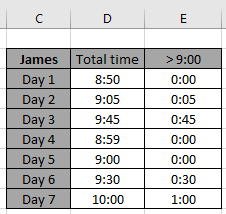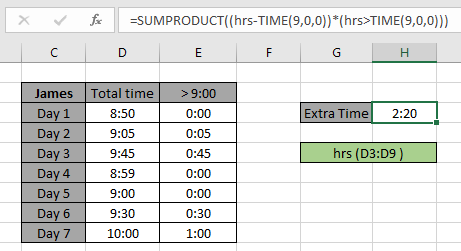SUM time over 9 hours in Excel

In this article, we will learn how to SUM time over 9 hours in Excel.

In simple words, while working with time data tables, sometimes we need to calculate time values from table based on a condition. Condition is to get the sum of time values which are above 9 hours.

For this article we will be needing the use the following functions:

Now we will make a formula out of these functions. Here we will given the time value data and we needed sum time values where value is greater than 9 hours.

Use the formula:

= SUMPRODUCT ( ( times - TIME (9,0,0) ) * ( times > TIME (9,0,0) ) )

variables:
times: array of Time values

Let's test this formula via running it on an example
Example:
James has to complete 9 hrs daily but he gets to use the extra time above 9 hrs in the next week.
We have the time record of him for the last 7 days. And for recalculation purpose, we mentioned the extra time for each day.Here hrs ( D3:D9 ) given in as using named range.

Now we will use the following formula to get the SUM of time for the Month1 in F3 cell.
Use the Formula:

= SUMPRODUCT ( ( hrs - TIME (9,0,0) ) * ( hrs > TIME (9,0,0) ) )

Explanation:

• TIME (9,0,0) returns the 9:00:00 time value.
• hrs > TIME (9,0,0) returns the TRUE & FALSE values.
• hrs - TIME (9,0,0) returns the difference of the actual time value and 9:00 time value
• SUMPRODUCT function returns the SUM of values given in as array to the function.As you can see the total extra time he has which he can utilize is 2 hrs and 20 min.

As you can see from the above formula the you can get total sum of time values having criteria.

Notes:

1. The formula only works with numbers.
2. The formula works only when there are no duplicates in the lookup table
3. The SUMPRODUCT function considers non - numeric values as 0s.
4. The SUMPRODUCT function considers logic value TRUE as 1 and False as 0.
5. The argument array must be of same length else the function

Hope this article about how to Return SUM time over 9 hours in Excel is explanatory. Find more articles on SUMPRODUCT functions here. Please share your query below in the comment box. We will assist you.

Related Articles

How to use the SUMPRODUCT function in Excel: Returns the SUM after multiplication of values in multiple arrays in excel.

SUM if date is between : Returns the SUM of values between given dates or period in excel.

Sum if date is greater than given date: Returns the SUM of values after the given date or period in excel.

2 Ways to Sum by Month in Excel: Returns the SUM of values within a given specific month in excel.

How to Sum Multiple Columns with Condition: Returns the SUM of values across multiple columns having condition in excel

Popular Articles

50 Excel Shortcut to Increase Your Productivity

Edit a dropdown list

Absolute reference in Excel

If with conditional formatting

If with wildcards

Vlookup by date

Convert Inches To Feet and Inches in Excel 2016

Join first and last name in excel

Count cells which match either A or B

Terms and Conditions of use

The applications/code on this site are distributed as is and without warranties or liability. In no event shall the owner of the copyrights, or the authors of the applications/code be liable for any loss of profit, any problems or any damage resulting from the use or evaluation of the applications/code.